Next: Centroid clustering Up: Hierarchical clustering Previous: Time complexity of HAC   Contents   Index

# Group-average agglomerative clustering

Group-average agglomerative clustering or GAAC (see Figure 17.3 , (d)) evaluates cluster quality based on all similarities between documents, thus avoiding the pitfalls of the single-link and complete-link criteria, which equate cluster similarity with the similarity of a single pair of documents. GAAC is also called group-average clustering and average-link clustering . GAAC computes the average similarity SIM-GA of all pairs of documents, including pairs from the same cluster. But self-similarities are not included in the average: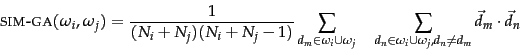(203)

where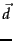is the length-normalized vector of document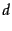,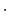denotes the dot product, and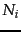and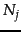are the number of documents in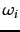and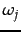, respectively.

The motivation for GAAC is that our goal in selecting two clustersandas the next merge in HAC is that the resulting merge cluster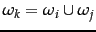should be coherent. To judge the coherence of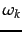, we need to look at all document-document similarities within, including those that occur withinand those that occur within.

We can compute the measure SIM-GA efficiently because the sum of individual vector similarities is equal to the similarities of their sums: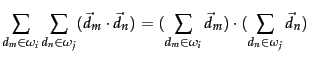(204)

With gatrick, we have: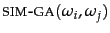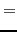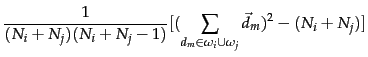(205)

The term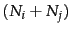on the right is the sum of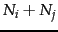self-similarities of value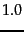. With this trick we can compute cluster similarity in constant time (assuming we have available the two vector sums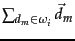and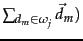instead of in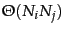. This is important because we need to be able to compute the function SIM on lines 18 and 20 in EFFICIENTHAC (Figure 17.8 ) in constant time for efficient implementations of GAAC. Note that for two singleton clusters, Equation 205 is equivalent to the dot product.

Equation 204 relies on the distributivity of the dot product with respect to vector addition. Since this is crucial for the efficient computation of a GAAC clustering, the method cannot be easily applied to representations of documents that are not real-valued vectors. Also, Equation 204 only holds for the dot product. While many algorithms introduced in this book have near-equivalent descriptions in terms of dot product, cosine similarity and Euclidean distance (cf. simdisfigs), Equation 204 can only be expressed using the dot product. This is a fundamental difference between single-link/complete-link clustering and GAAC. The first two only require a square matrix of similarities as input and do not care how these similarities were computed.

To summarize, GAAC requires (i) documents represented as vectors, (ii) length normalization of vectors, so that self-similarities are 1.0, and (iii) the dot product as the measure of similarity between vectors and sums of vectors.

The merge algorithms for GAAC and complete-link clustering are the same except that we use Equation 205 as similarity function in Figure 17.8 . Therefore, the overall time complexity of GAAC is the same as for complete-link clustering: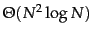. Like complete-link clustering, GAAC is not best-merge persistent (Exercise 17.10 ). This means that there is no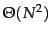algorithm for GAAC that would be analogous to thealgorithm for single-link in Figure 17.9 .

We can also define group-average similarity as including self-similarities: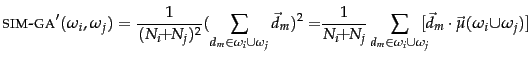(206)

where the centroid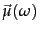is defined as in Equation 139 (page 139 ). This definition is equivalent to the intuitive definition of cluster quality as average similarity of documents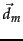to the cluster's centroid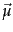.

Self-similarities are always equal to 1.0, the maximum possible value for length-normalized vectors. The proportion of self-similarities in Equation 206 is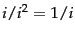for a cluster of size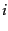. This gives an unfair advantage to small clusters since they will have proportionally more self-similarities. For two documents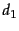,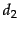with a similarity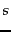, we have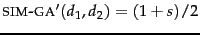. In contrast,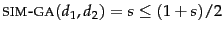. This similarity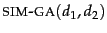of two documents is the same as in single-link, complete-link and centroid clustering. We prefer the definition in Equation 205, which excludes self-similarities from the average, because we do not want to penalize large clusters for their smaller proportion of self-similarities and because we want a consistent similarity valuefor document pairs in all four HAC algorithms.

Exercises.

• Apply group-average clustering to the points in and 17.7 . Map them onto the surface of the unit sphere in a three-dimensional space to get length-normalized vectors. Is the group-average clustering different from the single-link and complete-link clusterings?Next: Centroid clustering Up: Hierarchical clustering Previous: Time complexity of HAC   Contents   Index